This is “Atomic Spectra and Models of the Atom”, section 6.3 from the book Principles of General Chemistry (v. 1.0M). For details on it (including licensing), click here.

Has this book helped you? Consider passing it on:
Creative Commons supports free culture from music to education. Their licenses helped make this book available to you.
DonorsChoose.org helps people like you help teachers fund their classroom projects, from art supplies to books to calculators.

## 6.3 Atomic Spectra and Models of the Atom

### Learning Objective

1. To know the relationship between atomic spectra and the electronic structure of atoms.

The photoelectric effect provided indisputable evidence for the existence of the photon and thus the particle-like behavior of electromagnetic radiation. The concept of the photon, however, emerged from experimentation with thermal radiation, electromagnetic radiation emitted as the result of a source’s temperature, which produces a continuous spectrum of energies. More direct evidence was needed to verify the quantized nature of electromagnetic radiation. In this section, we describe how experimentation with visible light provided this evidence.

## Line Spectra

Although objects at high temperature emit a continuous spectrum of electromagnetic radiation (Figure 6.6 "Relationship between the Temperature of an Object and the Spectrum of Blackbody Radiation It Emits"), a different kind of spectrum is observed when pure samples of individual elements are heated. For example, when a high-voltage electrical discharge is passed through a sample of hydrogen gas at low pressure, the resulting individual isolated hydrogen atoms caused by the dissociation of H2 emit a red light. Unlike blackbody radiation, the color of the light emitted by the hydrogen atoms does not depend greatly on the temperature of the gas in the tube. When the emitted light is passed through a prism, only a few narrow lines, called a line spectrumA spectrum in which light of only a certain wavelength is emitted or absorbed, rather than a continuous range of wavelengths., are seen (Figure 6.9 "The Emission of Light by Hydrogen Atoms"), rather than a continuous range of colors. The light emitted by hydrogen atoms is red because, of its four characteristic lines, the most intense line in its spectrum is in the red portion of the visible spectrum, at 656 nm. With sodium, however, we observe a yellow color because the most intense lines in its spectrum are in the yellow portion of the spectrum, at about 589 nm.

Figure 6.9 The Emission of Light by Hydrogen Atoms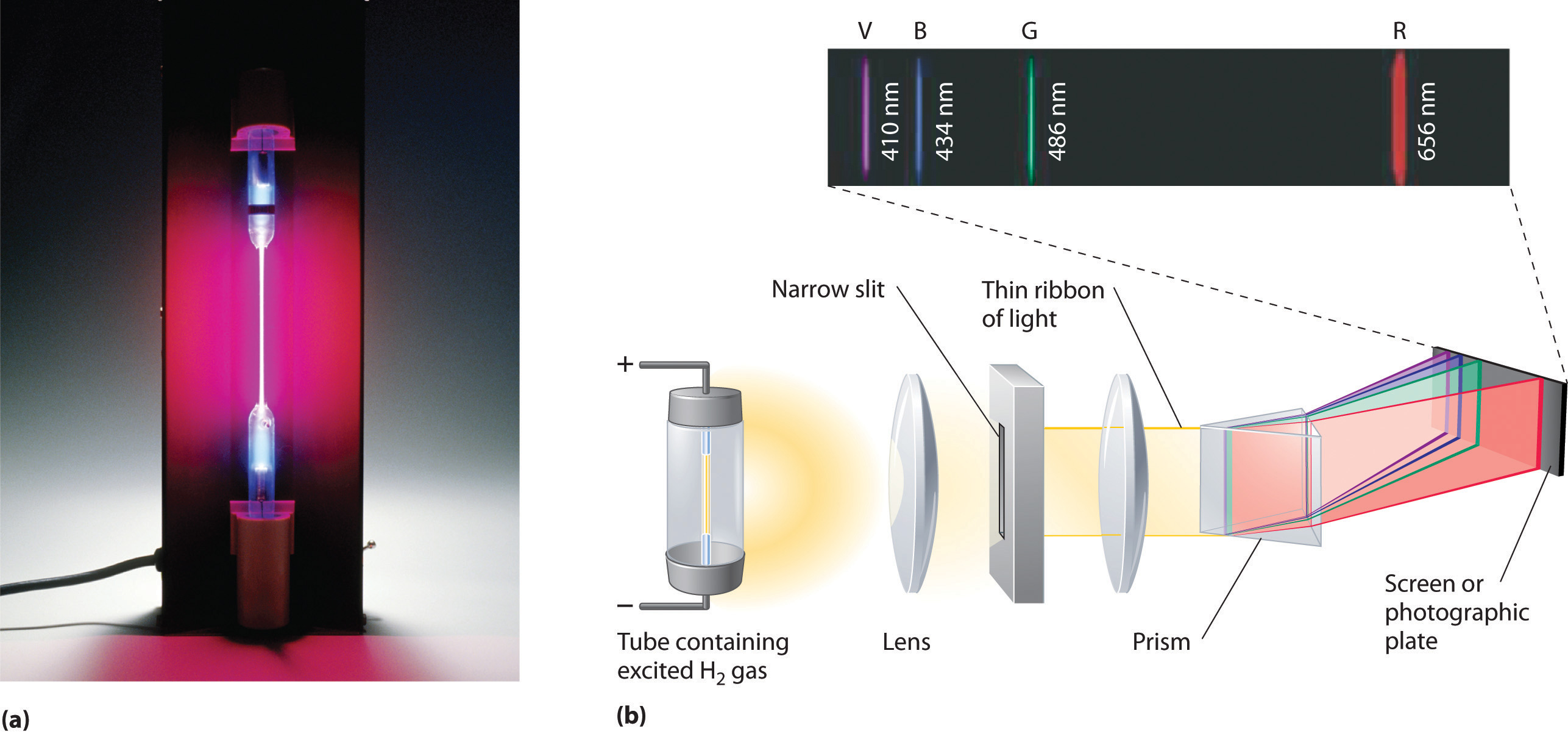(a) A sample of excited hydrogen atoms emits a characteristic red light. (b) When the light emitted by a sample of excited hydrogen atoms is split into its component wavelengths by a prism, four characteristic violet, blue, green, and red emission lines can be observed, the most intense of which is at 656 nm.

Such emission spectra were observed for many other elements in the late 19th century, which presented a major challenge because classical physics was unable to explain them. Part of the explanation is provided by Planck’s equation (Equation 6.5): the observation of only a few values of λ (or ν) in the line spectrum meant that only a few values of E were possible. Thus the energy levels of a hydrogen atom had to be quantized; in other words, only states that had certain values of energy were possible, or allowed. If a hydrogen atom could have any value of energy, then a continuous spectrum would have been observed, similar to blackbody radiation.

In 1885, a Swiss mathematics teacher, Johann Balmer (1825–1898), showed that the frequencies of the lines observed in the visible region of the spectrum of hydrogen fit a simple equation that can be expressed as follows:

Equation 6.7

where n = 3, 4, 5, 6. As a result, these lines are known as the Balmer series. The Swedish physicist Johannes Rydberg (1854–1919) subsequently restated and expanded Balmer’s result in the Rydberg equation:

Equation 6.8

$1 λ = ℛ ( 1 n 1 2 − 1 n 2 2 )$

where n1 and n2 are positive integers, n2 > n1, and $ℛ,$ the Rydberg constant, has a value of 1.09737 × 107 m−1.

### Johann Balmer (1825–1898)

A mathematics teacher at a secondary school for girls in Switzerland, Balmer was 60 years old when he wrote the paper on the spectral lines of hydrogen that made him famous. He published only one other paper on the topic, which appeared when he was 72 years old.

Like Balmer’s equation, Rydberg’s simple equation described the wavelengths of the visible lines in the emission spectrum of hydrogen (with n1 = 2, n2 = 3, 4, 5,…). More important, Rydberg’s equation also described the wavelengths of other series of lines that would be observed in the emission spectrum of hydrogen: one in the ultraviolet (n1 = 1, n2 = 2, 3, 4,…) and one in the infrared (n1 = 3, n2 = 4, 5, 6). Unfortunately, scientists had not yet developed any theoretical justification for an equation of this form.

## The Bohr Model

In 1913, a Danish physicist, Niels Bohr (1885–1962; Nobel Prize in Physics, 1922), proposed a theoretical model for the hydrogen atom that explained its emission spectrum. Bohr’s model required only one assumption: The electron moves around the nucleus in circular orbits that can have only certain allowed radii. As discussed in Chapter 1 "Introduction to Chemistry", Rutherford’s earlier model of the atom had also assumed that electrons moved in circular orbits around the nucleus and that the atom was held together by the electrostatic attraction between the positively charged nucleus and the negatively charged electron. Although we now know that the assumption of circular orbits was incorrect, Bohr’s insight was to propose that the electron could occupy only certain regions of space.

### Niels Bohr (1885–1962)

During the Nazi occupation of Denmark in World War II, Bohr escaped to the United States, where he became associated with the Atomic Energy Project. In his final years, he devoted himself to the peaceful application of atomic physics and to resolving political problems arising from the development of atomic weapons.

Using classical physics, Bohr showed that the energy of an electron in a particular orbit is given by

Equation 6.9

$E n = − ℛ h c n 2$

where $ℛ$ is the Rydberg constant, h is Planck’s constant, c is the speed of light, and n is a positive integer corresponding to the number assigned to the orbit, with n = 1 corresponding to the orbit closest to the nucleus.The negative sign in Equation 6.9 is a convention indicating that the electron-nucleus pair has a lower energy when they are near each other than when they are infinitely far apart, corresponding to n = ∞. The latter condition is arbitrarily assigned an energy of zero. Thus the orbit with n = 1 is the lowest in energy. Because a hydrogen atom with its one electron in this orbit has the lowest possible energy, this is the ground stateThe most stable arrangement of electrons for an element or a compound., the most stable arrangement for a hydrogen atom. As n increases, the radius of the orbit increases; the electron is farther from the proton, which results in a less stable arrangement with higher potential energy (Figure 6.10 "The Bohr Model of the Hydrogen Atom"). A hydrogen atom with an electron in an orbit with n > 1 is therefore in an excited stateAny arrangement of electrons that is higher in energy than the ground state.: its energy is higher than the energy of the ground state. When an atom in an excited state undergoes a transition to the ground state in a process called decay, it loses energy by emitting a photon whose energy corresponds to the difference in energy between the two states (Figure 6.11 "The Emission of Light by a Hydrogen Atom in an Excited State").

Figure 6.10 The Bohr Model of the Hydrogen Atom(a) The distance of the orbit from the nucleus increases with increasing n. (b) The energy of the orbit becomes increasingly less negative with increasing n.

Figure 6.11 The Emission of Light by a Hydrogen Atom in an Excited State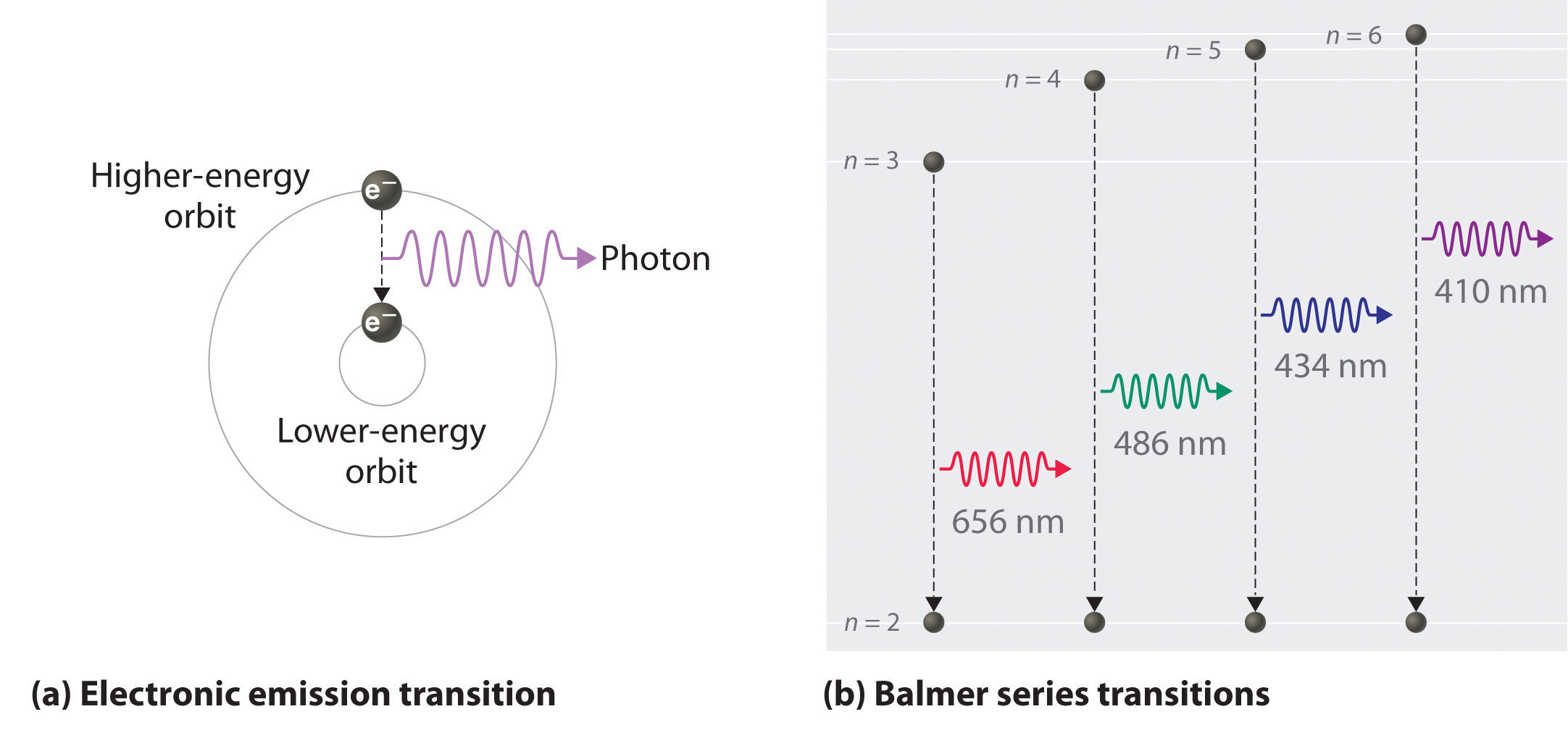(a) Light is emitted when the electron undergoes a transition from an orbit with a higher value of n (at a higher energy) to an orbit with a lower value of n (at lower energy). (b) The Balmer series of emission lines is due to transitions from orbits with n ≥ 3 to the orbit with n = 2. The differences in energy between these levels corresponds to light in the visible portion of the electromagnetic spectrum.

So the difference in energy (ΔE) between any two orbits or energy levels is given by $ΔE=En1−En2,$ where n1 is the final orbit and n2 the initial orbit. Substituting from Bohr’s equation (Equation 6.9) for each energy value gives

Equation 6.10

$Δ E = E final − E initial = − ℛ h c n 1 2 − ( − ℛ h c n 2 2 ) = − ℛ h c ( 1 n 1 2 − 1 n 2 2 )$

If n2 > n1, the transition is from a higher energy state (larger-radius orbit) to a lower energy state (smaller-radius orbit), as shown by the dashed arrow in part (a) in Figure 6.11 "The Emission of Light by a Hydrogen Atom in an Excited State". Substituting hc/λ for ΔE gives

Equation 6.11

$Δ E = h c λ = − ℛ h c ( 1 n 1 2 − 1 n 2 2 )$

Canceling hc on both sides gives

Equation 6.12

$1 λ = − ℛ ( 1 n 1 2 − 1 n 2 2 )$

Except for the negative sign, this is the same equation that Rydberg obtained experimentally. The negative sign in Equation 6.11 and Equation 6.12 indicates that energy is released as the electron moves from orbit n2 to orbit n1 because orbit n2 is at a higher energy than orbit n1. Bohr calculated the value of $ℛ$ independently and obtained a value of 1.0974 × 107 m−1, the same number Rydberg had obtained by analyzing the emission spectra.

We can now understand the physical basis for the Balmer series of lines in the emission spectrum of hydrogen (part (b) in Figure 6.9 "The Emission of Light by Hydrogen Atoms"). As shown in part (b) in Figure 6.11 "The Emission of Light by a Hydrogen Atom in an Excited State", the lines in this series correspond to transitions from higher-energy orbits (n > 2) to the second orbit (n = 2). Thus the hydrogen atoms in the sample have absorbed energy from the electrical discharge and decayed from a higher-energy excited state (n > 2) to a lower-energy state (n = 2) by emitting a photon of electromagnetic radiation whose energy corresponds exactly to the difference in energy between the two states (part (a) in Figure 6.11 "The Emission of Light by a Hydrogen Atom in an Excited State"). The n = 3 to n = 2 transition gives rise to the line at 656 nm (red), the n = 4 to n = 2 transition to the line at 486 nm (green), the n = 5 to n = 2 transition to the line at 434 nm (blue), and the n = 6 to n = 2 transition to the line at 410 nm (violet). Because a sample of hydrogen contains a large number of atoms, the intensity of the various lines in a line spectrum depends on the number of atoms in each excited state. At the temperature in the gas discharge tube, more atoms are in the n = 3 than the n ≥ 4 levels. Consequently, the n = 3 to n = 2 transition is the most intense line, producing the characteristic red color of a hydrogen discharge (part (a) in Figure 6.9 "The Emission of Light by Hydrogen Atoms"). Other families of lines are produced by transitions from excited states with n > 1 to the orbit with n = 1 or to orbits with n ≥ 3. These transitions are shown schematically in Figure 6.12 "Electron Transitions Responsible for the Various Series of Lines Observed in the Emission Spectrum of Hydrogen".

Figure 6.12 Electron Transitions Responsible for the Various Series of Lines Observed in the Emission Spectrum of Hydrogen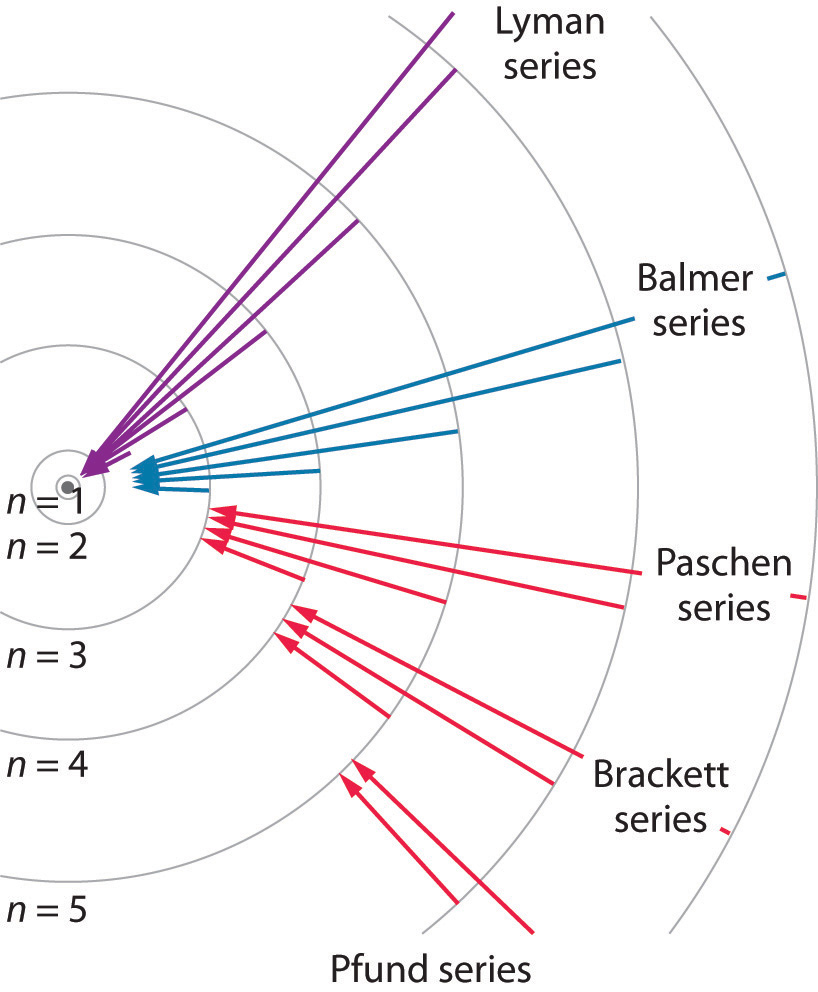The Lyman series of lines is due to transitions from higher-energy orbits to the lowest-energy orbit (n = 1); these transitions release a great deal of energy, corresponding to radiation in the ultraviolet portion of the electromagnetic spectrum. The Paschen, Brackett, and Pfund series of lines are due to transitions from higher-energy orbits to orbits with n = 3, 4, and 5, respectively; these transitions release substantially less energy, corresponding to infrared radiation. (Orbits are not drawn to scale.)

In contemporary applications, electron transitions are used in timekeeping that needs to be exact. Telecommunications systems, such as cell phones, depend on timing signals that are accurate to within a millionth of a second per day, as are the devices that control the US power grid. Global positioning system (GPS) signals must be accurate to within a billionth of a second per day, which is equivalent to gaining or losing no more than one second in 1,400,000 years. Quantifying time requires finding an event with an interval that repeats on a regular basis. To achieve the accuracy required for modern purposes, physicists have turned to the atom. The current standard used to calibrate clocks is the cesium atom. Supercooled cesium atoms are placed in a vacuum chamber and bombarded with microwaves whose frequencies are carefully controlled. When the frequency is exactly right, the atoms absorb enough energy to undergo an electronic transition to a higher-energy state. Decay to a lower-energy state emits radiation. The microwave frequency is continually adjusted, serving as the clock’s pendulum. In 1967, the second was defined as the duration of 9,192,631,770 oscillations of the resonant frequency of a cesium atom, called the cesium clock. Research is currently under way to develop the next generation of atomic clocks that promise to be even more accurate. Such devices would allow scientists to monitor vanishingly faint electromagnetic signals produced by nerve pathways in the brain and geologists to measure variations in gravitational fields, which cause fluctuations in time, that would aid in the discovery of oil or minerals.

### Example 3

The so-called Lyman series of lines in the emission spectrum of hydrogen corresponds to transitions from various excited states to the n = 1 orbit. Calculate the wavelength of the lowest-energy line in the Lyman series to three significant figures. In what region of the electromagnetic spectrum does it occur?

Given: lowest-energy orbit in the Lyman series

Asked for: wavelength of the lowest-energy Lyman line and corresponding region of the spectrum

Strategy:

A Substitute the appropriate values into Equation 6.8 (the Rydberg equation) and solve for λ.

B Use Figure 6.4 "The Electromagnetic Spectrum" to locate the region of the electromagnetic spectrum corresponding to the calculated wavelength.

Solution:

We can use the Rydberg equation to calculate the wavelength:

$1 λ = ℛ ( 1 n 1 2 − 1 n 2 2 )$

A For the Lyman series, n1 = 1. The lowest-energy line is due to a transition from the n = 2 to n = 1 orbit because they are the closest in energy.

$1 λ = ℛ ( 1 n 1 2 − 1 n 2 2 ) = 1.097 × 10 7 m − 1 ( 1 1 − 1 4 ) = 8.228 × 10 6 m − 1$

and

λ = 1.215 × 10−7 m = 122 nm

B This wavelength is in the ultraviolet region of the spectrum.

Exercise

The Pfund series of lines in the emission spectrum of hydrogen corresponds to transitions from higher excited states to the n = 5 orbit. Calculate the wavelength of the second line in the Pfund series to three significant figures. In which region of the spectrum does it lie?

Answer: 4.65 × 103 nm; infrared

Bohr’s model of the hydrogen atom gave an exact explanation for its observed emission spectrum. The following are his key contributions to our understanding of atomic structure:

• Electrons can occupy only certain regions of space, called orbits.
• Orbits closer to the nucleus are lower in energy.
• Electrons can move from one orbit to another by absorbing or emitting energy, giving rise to characteristic spectra.

Unfortunately, Bohr could not explain why the electron should be restricted to particular orbits. Also, despite a great deal of tinkering, such as assuming that orbits could be ellipses rather than circles, his model could not quantitatively explain the emission spectra of any element other than hydrogen (Figure 6.13 "The Emission Spectra of Elements Compared with Hydrogen"). In fact, Bohr’s model worked only for species that contained just one electron: H, He+, Li2+, and so forth. Scientists needed a fundamental change in their way of thinking about the electronic structure of atoms to advance beyond the Bohr model.

Figure 6.13 The Emission Spectra of Elements Compared with Hydrogen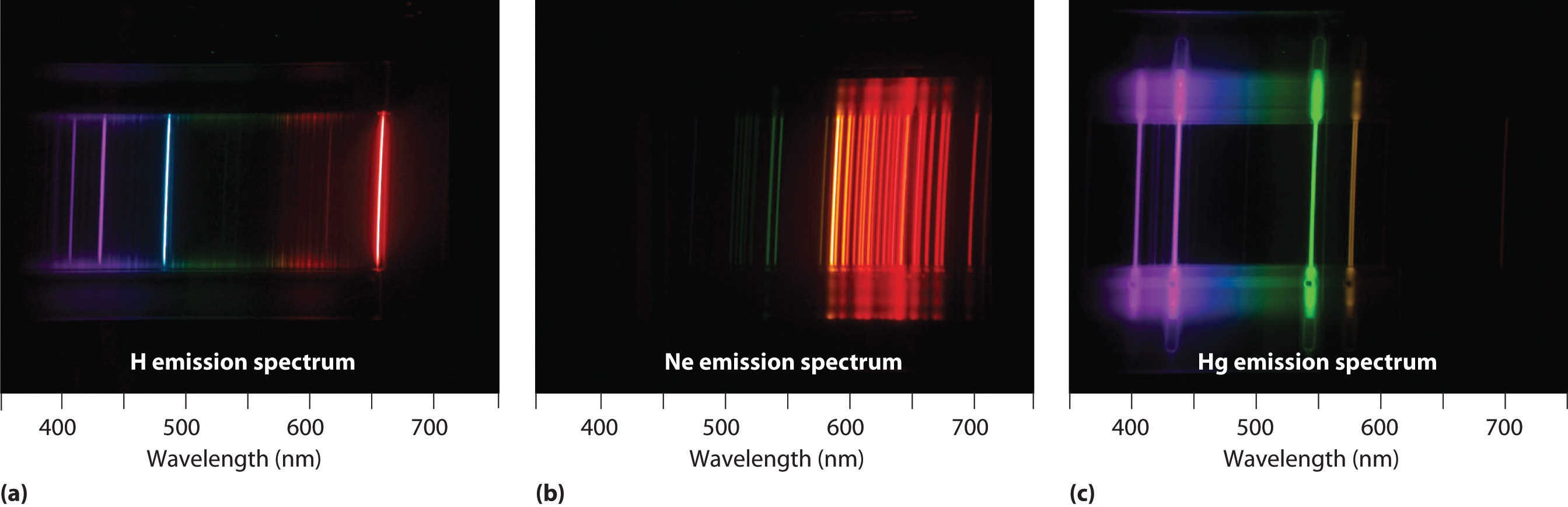These images show (a) hydrogen gas, which is atomized to hydrogen atoms in the discharge tube; (b) neon; and (c) mercury.

Thus far we have explicitly considered only the emission of light by atoms in excited states, which produces an emission spectrumA spectrum produced by the emission of light by atoms in excited states.. The converse, absorption of light by ground-state atoms to produce an excited state, can also occur, producing an absorption spectrumA spectrum produced by the absorption of light by ground-state atoms.. Because each element has characteristic emission and absorption spectra, scientists can use such spectra to analyze the composition of matter, as we describe in Section 6.4 "The Relationship between Energy and Mass".

### Note the Pattern

When an atom emits light, it decays to a lower energy state; when an atom absorbs light, it is excited to a higher energy state.

## Applications of Emission and Absorption Spectra

If white light is passed through a sample of hydrogen, hydrogen atoms absorb energy as an electron is excited to higher energy levels (orbits with n ≥ 2). If the light that emerges is passed through a prism, it forms a continuous spectrum with black lines (corresponding to no light passing through the sample) at 656, 468, 434, and 410 nm. These wavelengths correspond to the n = 2 to n = 3, n = 2 to n = 4, n = 2 to n = 5, and n = 2 to n = 6 transitions. Any given element therefore has both a characteristic emission spectrum and a characteristic absorption spectrum, which are essentially complementary images.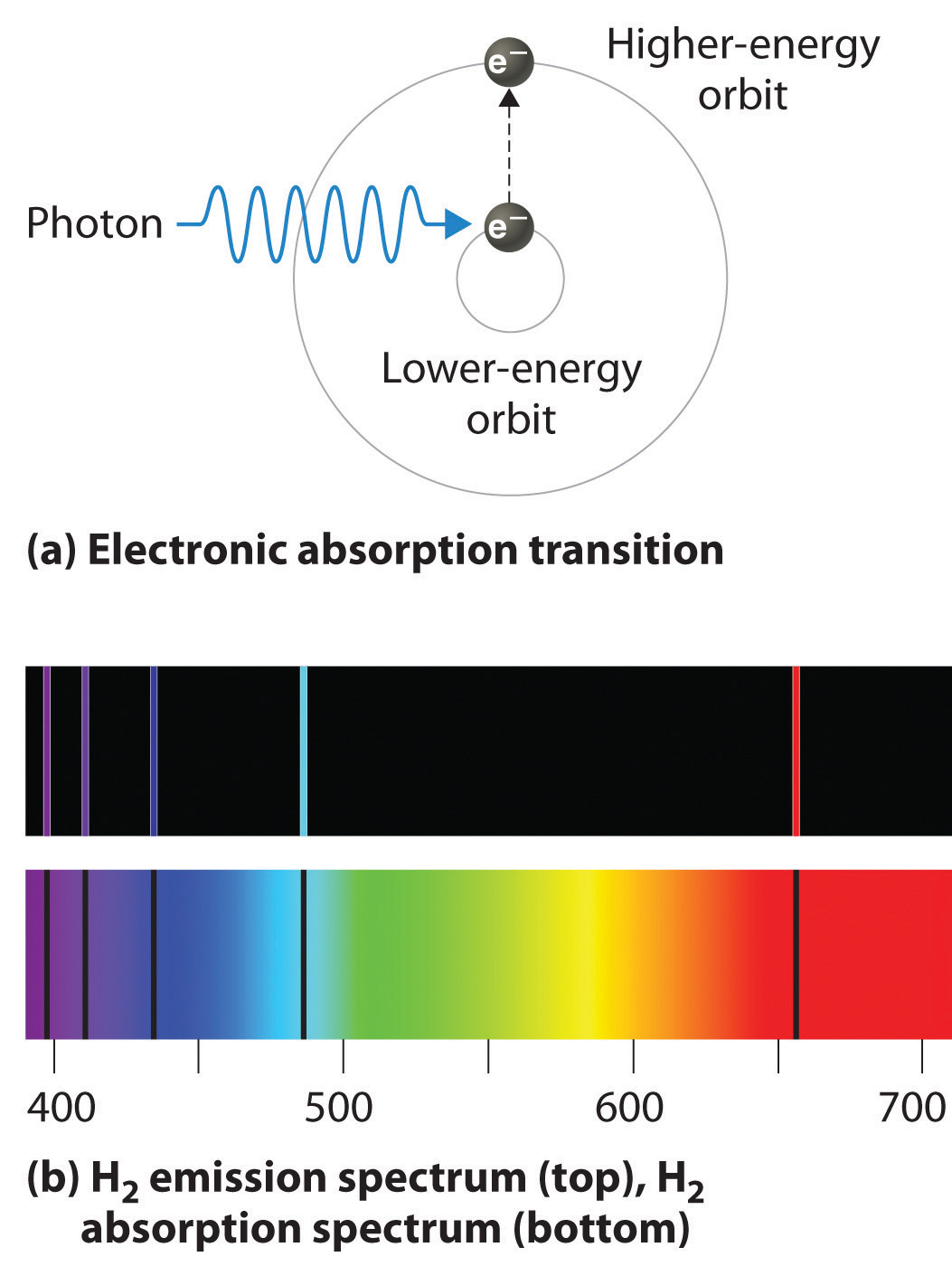Absorption of light by a hydrogen atom. (a) When a hydrogen atom absorbs a photon of light, an electron is excited to an orbit that has a higher energy and larger value of n. (b) Images of the emission and absorption spectra of hydrogen are shown here.

Emission and absorption spectra form the basis of spectroscopy, which uses spectra to provide information about the structure and the composition of a substance or an object. In particular, astronomers use emission and absorption spectra to determine the composition of stars and interstellar matter. As an example, consider the spectrum of sunlight shown in Figure 6.14 "The Visible Spectrum of Sunlight". Because the sun is very hot, the light it emits is in the form of a continuous emission spectrum. Superimposed on it, however, is a series of dark lines due primarily to the absorption of specific frequencies of light by cooler atoms in the outer atmosphere of the sun. By comparing these lines with the spectra of elements measured on Earth, we now know that the sun contains large amounts of hydrogen, iron, and carbon, along with smaller amounts of other elements. During the solar eclipse of 1868, the French astronomer Pierre Janssen (1824–1907) observed a set of lines that did not match those of any known element. He suggested that they were due to the presence of a new element, which he named helium, from the Greek helios, meaning “sun.” Helium was finally discovered in uranium ores on Earth in 1895.

Figure 6.14 The Visible Spectrum of Sunlight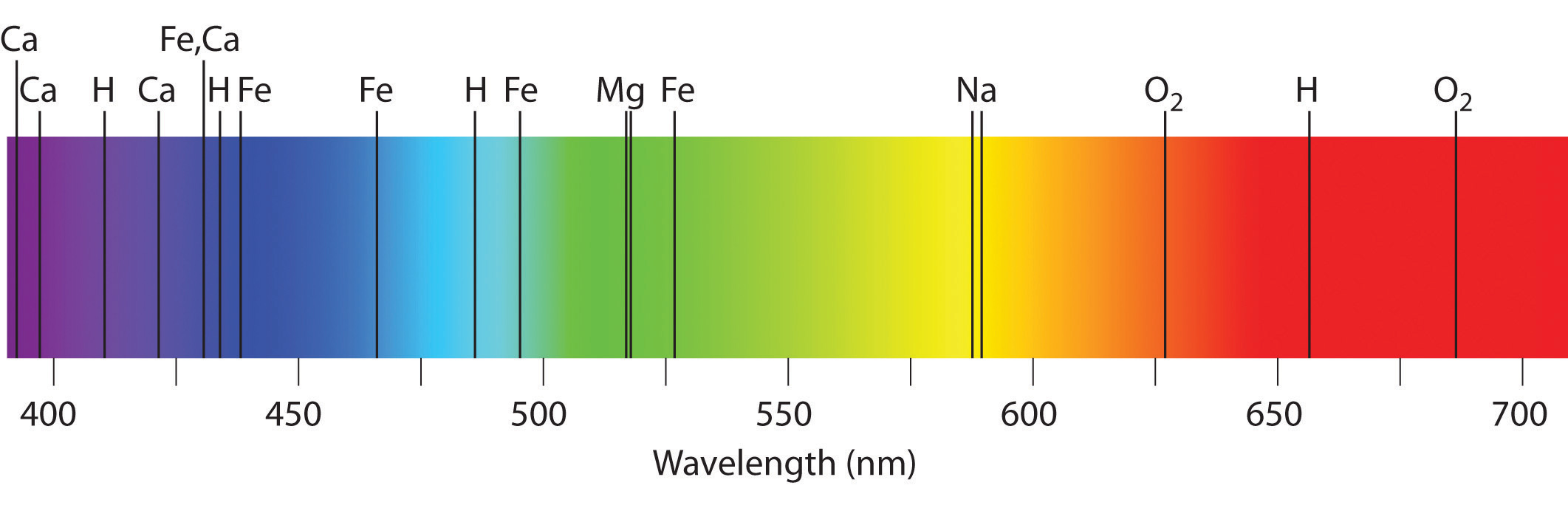The characteristic dark lines are mostly due to the absorption of light by elements that are present in the cooler outer part of the sun’s atmosphere; specific elements are indicated by the labels. The lines at 628 and 687 nm, however, are due to the absorption of light by oxygen molecules in Earth’s atmosphere.

The familiar red color of “neon” signs used in advertising is due to the emission spectrum of neon shown in part (b) in Figure 6.13 "The Emission Spectra of Elements Compared with Hydrogen". Similarly, the blue and yellow colors of certain street lights are caused, respectively, by mercury and sodium discharges. In all these cases, an electrical discharge excites neutral atoms to a higher energy state, and light is emitted when the atoms decay to the ground state. In the case of mercury, most of the emission lines are below 450 nm, which produces a blue light (part (c) in Figure 6.13 "The Emission Spectra of Elements Compared with Hydrogen"). In the case of sodium, the most intense emission lines are at 589 nm, which produces an intense yellow light.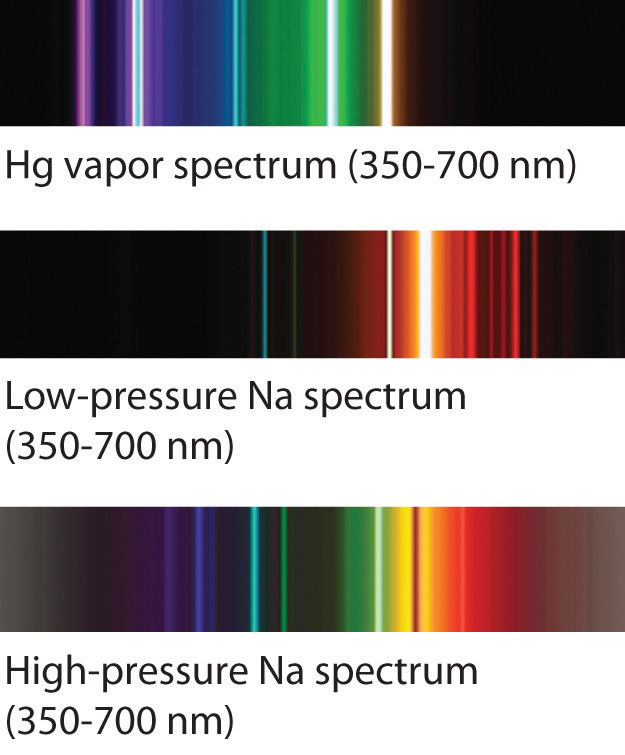Sodium and mercury spectra. Many street lights use bulbs that contain sodium or mercury vapor. Due to the very different emission spectra of these elements, they emit light of different colors.

## The Chemistry of Fireworks

Figure 6.15 The Chemistry of Fireworks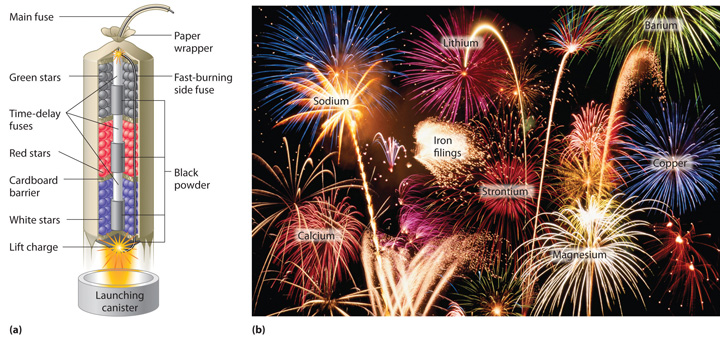(a) In the “multibreak” shell used for fireworks, the chambers contain mixtures of fuels and oxidizers plus compounds for special effects (“stars”) connected by time-delay fuses so that the chambers explode in stages. (b) The finale of a fireworks display usually consists of many shells fired simultaneously to give a dazzling multicolor display. The labels indicate the substances that are responsible for the colors of some of the fireworks shown.

The colors of fireworks are also due to atomic emission spectra. As shown in part (a) in Figure 6.15 "The Chemistry of Fireworks", a typical shell used in a fireworks display contains gunpowder to propel the shell into the air and a fuse to initiate a variety of redox reactions that produce heat and small explosions. Thermal energy excites the atoms to higher energy states; as they decay to lower energy states, the atoms emit light that gives the familiar colors. When oxidant/reductant mixtures listed in Table 6.2 "Common Chemicals Used in the Manufacture of Fireworks*" are ignited, a flash of white or yellow light is produced along with a loud bang. Achieving the colors shown in part (b) in Figure 6.15 "The Chemistry of Fireworks" requires adding a small amount of a substance that has an emission spectrum in the desired portion of the visible spectrum. For example, sodium is used for yellow because of its 589 nm emission lines. The intense yellow color of sodium would mask most other colors, so potassium and ammonium salts, rather than sodium salts, are usually used as oxidants to produce other colors, which explains the preponderance of such salts in Table 6.2 "Common Chemicals Used in the Manufacture of Fireworks*". Strontium salts, which are also used in highway flares, emit red light, whereas barium gives a green color. Blue is one of the most difficult colors to achieve. Copper(II) salts emit a pale blue light, but copper is dangerous to use because it forms highly unstable explosive compounds with anions such as chlorate. As you might guess, preparing fireworks with the desired properties is a complex, challenging, and potentially hazardous process.

Table 6.2 Common Chemicals Used in the Manufacture of Fireworks*

Oxidizers Fuels (reductants) Special effects
ammonium perchlorate aluminum blue flame: copper carbonate, copper sulfate, or copper oxide
barium chlorate antimony sulfide red flame: strontium nitrate or strontium carbonate
barium nitrate charcoal white flame: magnesium or aluminum
potassium chlorate magnesium yellow flame: sodium oxalate or cryolite (Na3AlF6)
potassium nitrate sulfur green flame: barium nitrate or barium chlorate
potassium perchlorate titanium white smoke: potassium nitrate plus sulfur
strontium nitrate colored smoke: potassium chlorate and sulfur, plus organic dye
whistling noise: potassium benzoate or sodium salicylate
white sparks: aluminum, magnesium, or titanium
gold sparks: iron fillings or charcoal
*Almost any combination of an oxidizer and a fuel may be used along with the compounds needed to produce a desired special effect.

## Lasers

Most light emitted by atoms is polychromatic—containing more than one wavelength. In contrast, lasers (from light amplification by stimulated emission of radiation) emit monochromatic light—a single wavelength only. Lasers have many applications in fiber-optic telecommunications, the reading and recording of compact discs (CDs) and digital video discs (DVDs), steel cutting, and supermarket checkout scanners. Laser beams are generated by the same general phenomenon that gives rise to emission spectra, with one difference: only a single excited state is produced, which in principle results in only a single frequency of emitted light. In practice, however, inexpensive commercial lasers actually emit light with a very narrow range of wavelengths.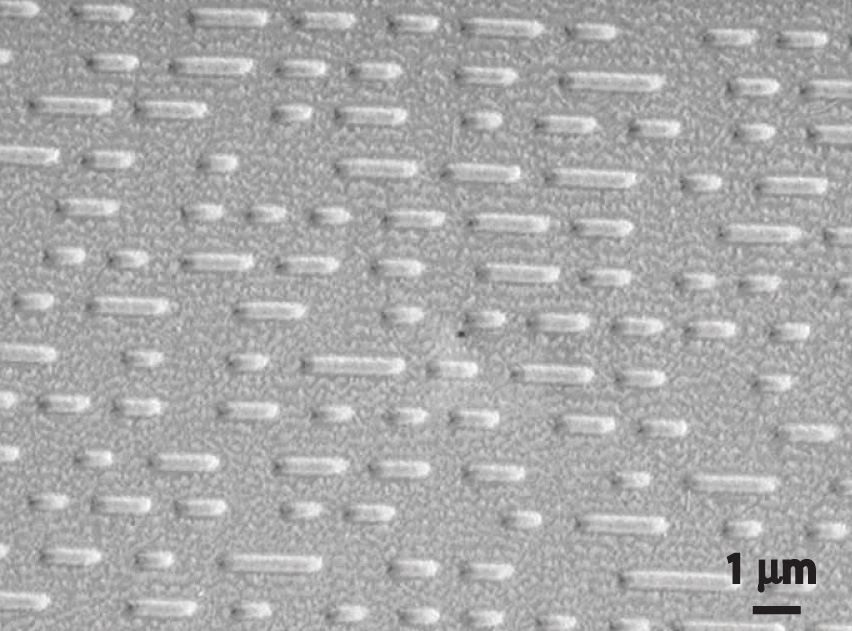How a CD player uses a laser to read a CD. Inside a CD is a flat, light-reflecting layer called “land.” On the land are many “pits” recorded in a spiral-shaped track. (From the laser’s point of view, pits are actually the “bumps” shown here because the master disc with pits is duplicated negatively, turning the pits into bumps.) Pits have the same light-reflecting surface as land, but there are differences in the frequencies of the reflected light in the pit and the land, making light reflected by pits relatively dark compared with light reflected by land.

The operation of a ruby laser, the first type of laser used commercially, is shown schematically in Figure 6.16 "A Ruby Laser". Ruby is an impure form of aluminum oxide (Al2O3) in which Cr3+ replaces some of the Al3+ ions. The red color of the gem is caused by the absorption of light in the blue region of the visible spectrum by Cr3+ ions, which leaves only the longer wavelengths to be reflected back to the eye. One end of a ruby bar is coated with a fully reflecting mirror, and the mirror on the other end is only partially reflecting. When flashes of white light from a flash lamp excite the Cr3+ ions, they initially decay to a relatively long-lived excited state and can subsequently decay to the ground state by emitting a photon of red light. Some of these photons are reflected back and forth by the mirrored surfaces. As shown in part (b) in Figure 6.16 "A Ruby Laser", each time a photon interacts with an excited Cr3+ ion, it can stimulate that ion to emit another photon that has the same wavelength and is synchronized (in phase) with the first wave. This process produces a cascade of photons traveling back and forth, until the intense beam emerges through the partially reflecting mirror. Ruby is only one substance that is used to produce a laser; the choice of material determines the wavelength of light emitted, from infrared to ultraviolet, and the light output can be either continuous or pulsed.

Figure 6.16 A Ruby Laser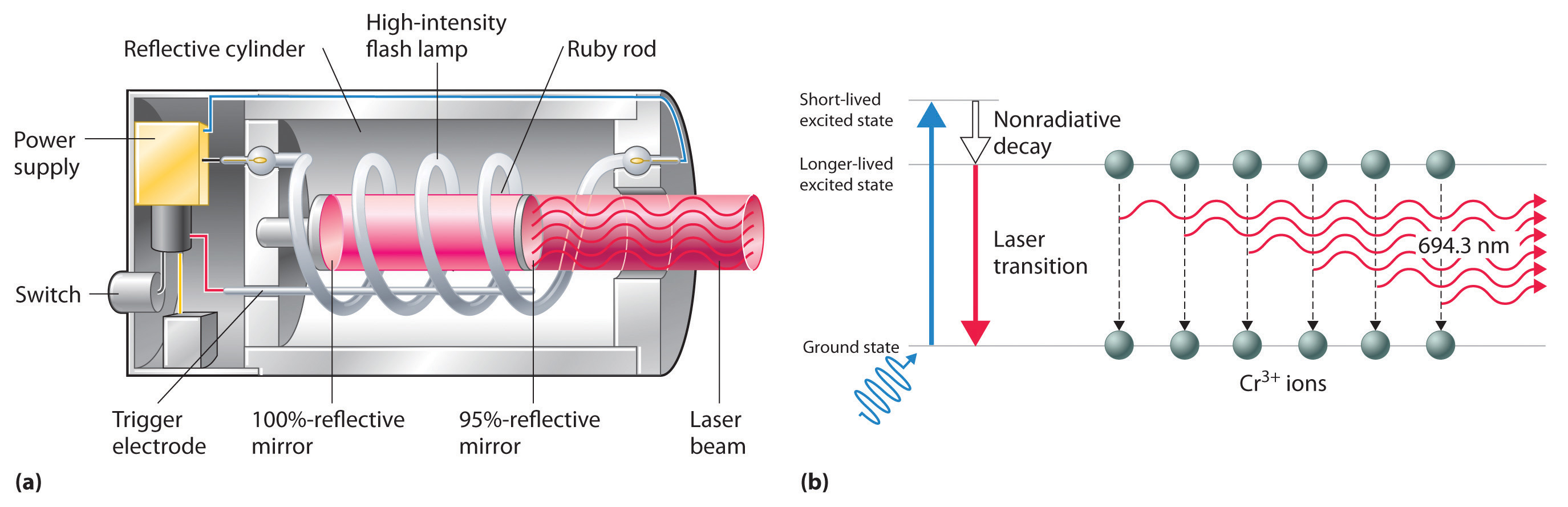(a) This cutaway view of a ruby laser shows the ruby rod, the flash lamp used to excite the Cr3+ ions in the ruby, and the totally and partially reflective mirrors. (b) This schematic drawing illustrates how light from the flash lamp excites the Cr3+ ions to a short-lived excited state, which is followed by decay to a longer-lived excited state that is responsible for the stimulated in-phase emission of light by the laser.

When used in a DVD player or a CD player, light emitted by a laser passes through a transparent layer of plastic on the CD and is reflected by an underlying aluminum layer, which contains pits or flat regions that were created when the CD was recorded. Differences in the frequencies of the transmitted and reflected light are detected by light-sensitive equipment that converts these differences into binary code, a series of 1s and 0s, which is translated electronically into recognizable sounds and images.

### Key Equation

Rydberg equation

Equation 6.8: $1λ=ℛ(1n12−1n22)$

### Summary

Atoms of individual elements emit light at only specific wavelengths, producing a line spectrum rather than the continuous spectrum of all wavelengths produced by a hot object. Niels Bohr explained the line spectrum of the hydrogen atom by assuming that the electron moved in circular orbits and that orbits with only certain radii were allowed. Lines in the spectrum were due to transitions in which an electron moved from a higher-energy orbit with a larger radius to a lower-energy orbit with smaller radius. The orbit closest to the nucleus represented the ground state of the atom and was most stable; orbits farther away were higher-energy excited states. Transitions from an excited state to a lower-energy state resulted in the emission of light with only a limited number of wavelengths. Bohr’s model could not, however, explain the spectra of atoms heavier than hydrogen.

Most light is polychromatic and contains light of many wavelengths. Light that has only a single wavelength is monochromatic and is produced by devices called lasers, which use transitions between two atomic energy levels to produce light in a very narrow range of wavelengths. Atoms can also absorb light of certain energies, resulting in a transition from the ground state or a lower-energy excited state to a higher-energy excited state. This produces an absorption spectrum, which has dark lines in the same position as the bright lines in the emission spectrum of an element.

### Key Takeaway

• There is an intimate connection between the atomic structure of an atom and its spectral characteristics.

### Conceptual Problems

1. Is the spectrum of the light emitted by isolated atoms of an element discrete or continuous? How do these spectra differ from those obtained by heating a bulk sample of a solid element? Explain your answers.

2. Explain why each element has a characteristic emission and absorption spectra. If spectral emissions had been found to be continuous rather than discrete, what would have been the implications for Bohr’s model of the atom?

3. Explain the differences between a ground state and an excited state. Describe what happens in the spectrum of a species when an electron moves from a ground state to an excited state. What happens in the spectrum when the electron falls from an excited state to a ground state?

4. What phenomenon causes a neon sign to have a characteristic color? If the emission spectrum of an element is constant, why do some neon signs have more than one color?

5. How is light from a laser different from the light emitted by a light source such as a light bulb? Describe how a laser produces light.

### Numerical Problems

1. Using a Bohr model and the transition from n = 2 to n = 3 in an atom with a single electron, describe the mathematical relationship between an emission spectrum and an absorption spectrum. What is the energy of this transition? What does the sign of the energy value represent in this case? What range of light is associated with this transition?

2. If a hydrogen atom is excited from an n = 1 state to an n = 3 state, how much energy does this correspond to? Is this an absorption or an emission? What is the wavelength of the photon involved in this process? To what region of the electromagnetic spectrum does this correspond?

3. The hydrogen atom emits a photon with a 486 nm wavelength, corresponding to an electron decaying from the n = 4 level to which level? What is the color of the emission?

4. An electron in a hydrogen atom can decay from the n = 3 level to n = 2 level. What is the color of the emitted light? What is the energy of this transition?

5. Calculate the wavelength and energy of the photon that gives rise to the third line in order of increasing energy in the Lyman series in the emission spectrum of hydrogen. In what region of the spectrum does this wavelength occur? Describe qualitatively what the absorption spectrum looks like.

6. The wavelength of one of the lines in the Lyman series of hydrogen is 121 nm. In what region of the spectrum does this occur? To which electronic transition does this correspond?

7. The emission spectrum of helium is shown. What change in energy (ΔE) in kilojoules per mole gives rise to each line?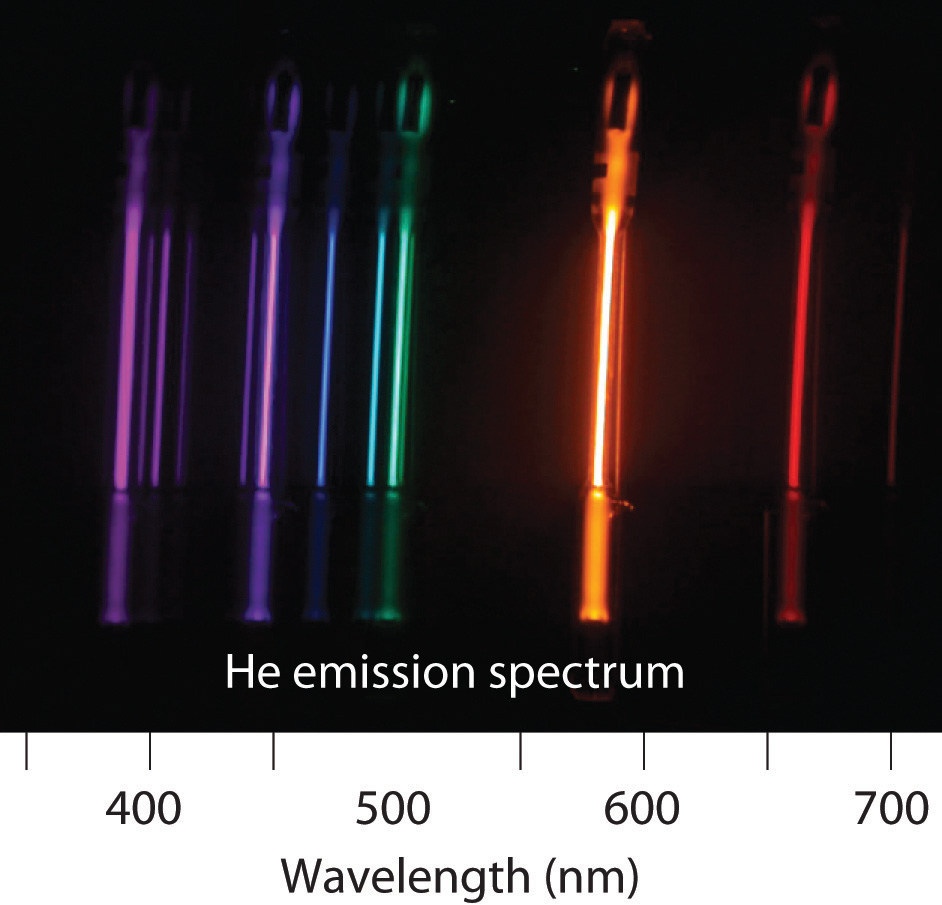8. Removing an electron from solid potassium requires 222 kJ/mol. Would you expect to observe a photoelectric effect for potassium using a photon of blue light (λ = 485 nm)? What is the longest wavelength of energy capable of ejecting an electron from potassium? What is the corresponding color of light of this wavelength?

9. The binding energy of an electron is the energy needed to remove an electron from its lowest energy state. According to Bohr’s postulates, calculate the binding energy of an electron in a hydrogen atom in kilojoules per mole. What wavelength in nanometers is required to remove such an electron?

10. As a radio astronomer, you have observed spectral lines for hydrogen corresponding to a state with n = 320, and you would like to produce these lines in the laboratory. Is this feasible? Why or why not?